top of page

#### A Beginners Guide to Bayesian Model Selection (Hypothesis Testing)

• What is Bayesian hypothesis testing?

• How are models used in Bayesian hypothesis testing?

• How are hypothesis tests related to measurements?

• What is the model prior?

• How do we solve a simple example of a Bayesian hypothesis test?

What is Bayesian hypothesis testing?

Bayesian hypothesis testing is called 'model comparison' or 'model selection', because the goal is to select the best model (hypothesis) from among the set of viable models that might plausibly explain a particular behavior, neural response, etc.

• In big-picture terms, this means computing the probabilities of each competing hypothesis to determine if one stands out as providing the best description/explanation of your experimental data.

• The first step is to define the hypotheses we wish to compare

• We must define at least two competing hypotheses
• If there is only a single viable hypothesis to explain some phenomenon, it is also (by definition) the best hypothesis - there is nothing to test

• In such cases, we can either
• make measurements based on this hypothesis
• or develop new competing hypotheses so that hypothesis testing can be done

• After collecting experimental data, we want to compute the evidence provided by our experimental data that either supports or deprecates the various competing hypotheses

• This evidence is based on computing the probabilities of the competing hypotheses
• these probabilities encode how well each hypothesis predicts the experimental data
• The essence of hypothesis testing is that the data we observe experimentally will be more consistent with the mechanisms and predictions of one hypothesis over the alternatives

• we assess the match between observed data and the predictions of alternative hypotheses via probabilities.

• By contrast, we show elsewhere that frequentist statistical hypothesis testing conflates measurement and hypothesis testing, by using statistical estimators in place of computing the probabilities of hypotheses

How are models used in Bayesian hypothesis testing?

As a general rule, when we analyze scientific data, our goal is usually to compare hypotheses.

We want to know if the data contain evidence to support particular hypothesis about the mechanisms underlying a given phenomenon (e.g., a behavioral change following stroke).

• Hypotheses are word-based descriptions of the mechanisms and causal networks we believe are at play in producing the behavior of interest.

• However, models are mathematical descriptions of those mechanisms and networks that we can use to generate testable predictions

• Hypothesis testing involves making predictions about the relationship between observable behavioral data, and unobservable neural mechanisms, computations, or parameters that produce or influence behavior.

• When we say we are testing hypotheses, this is a shorthand way of saying that we want to collect data and compare the predictions of competing models to determine which is more likely to be correct.

One of the simplest sets of models that we might wish to compare involves testing whether:

: body temperature,    , is normal (    = 98.6˚F)

: body temperature,    , is above-normal (    > 98.6˚F)

To do so, we start by constructing the observation model for thermometer observations:

which says that the i   thermometer observation is a noisy copy of the true temperature,    ,

• the i  observation is corrupted by the noise sample    .

Next we must address the distribution of noise samples

• The typical first guess for a noise model is the Gaussian distribution

• This guess can be refined later, if desired

The Gaussian noise model is:

which expands to:

by substituting the observation model.

Now, we use the observation and noise models to compute probabilities of the two competing hypotheses. To define these probabilities, we start with Bayes’ theorem:

Of the terms in this equation, we can assign all but           . However, since it does not include any elements with the i subscript (i.e., it is the same across hypotheses), we can eliminate it if we give the results of our analysis in terms of the odds ratio:

or, equivalently, in terms of evidence

which just transforms the odds ratio into a signal-to-noise ratio in decibels (db), a commonly-used unit for reporting the strength of a noisy signal (e.g., sound intensity) in scientific settings.

As a signal-to-noise measure, evidence also serves as an effect size that can be compared across domains. For example in the domain of sounds, 3db (odds ratio of about 2:1) corresponds to a bird softly chirping in the distance or leaves softly rustling. This is a sound intensity reliably above the quietest possible value of ‘no sound’: it is soft but clearly detectable, and we use this as a lowest threshold for a ‘real’ effect. This threshold is higher than the sensory threshold for detecting any sound at all (at about 1db), but lower than the sound level of a quiet conversation at about 10db or a jackhammer at about 100db. So while evidence that exceeds the 3db lowest threshold will point you toward preferring (i.e,. ‘quiet evidence’) one hypothesis over the alternatives, 100db should be considered an overwhelming level of evidence (very ‘loud’ evidence) favoring one hypothesis over alternatives.

How are hypothesis tests related to measurements?

The next step in completing the hypothesis test and computing the evidence favoring     , [i.e., the evidence         ], is to compute the likelihood terms,                , for all indices i

This likelihood term may look familiar from our discussion of measurement.

When computing the posterior distribution used in a measurement, Bayes’ theorem is written:

where the term in the denominator on the right,              , is the ‘model likelihood’.  This term serves as the normalization constant for the measurement equation,                         , and is therefore equal to:

What is the model prior?

The model likelihood is one of the two terms required to compute evidence. Recall that the other term is the model prior,            , from the proportional relation:

This model prior encodes the level of support favoring each of the competing models, prior to having observed the current experimental data.

Although the two models we define here do not cover all physically possible body temperatures, if they are the only viable models to be considered, we can assign model prior probabilities of:

Further, if we do not wish to favor either of the models prior to observing experimental data, we must assign

.

If we had information that would cause us to have a preference for one model over the other, we could explore different assignments to the model priors.

How do we solve a simple example of a Bayesian hypothesis test?

Signal detection example:

How do we complete the body temperature hypothesis test?

First, we require a dataset. Let’s say our thermometer readings are: x = [99.3, 98.7, 99.1].

To make the computations, we start from the proportional relation:

Given that the model priors are equal (and therefore don't influence the final result), we only need compute the two model likelihoods (indexed by i), which are defined by:

To simplify our computations and focus on the method of solving the problem, let’s assume that we know the noise power in the observation model, and set            (i.e., 1 degree).

For the normal-temperature model, this means that all parameters are known, and the 2d prior becomes a discrete probability function with all mass located at the point (98.6, 1) in           space.

The 2d likelihood reduces to a 1d likelihood that cuts through the           point on the    -axis, and can be plotted as a 1d function (Fig. 1a).

The model likelihood is the integral of the product of this likelihood and the 2d prior over          with its probability mass located entirely at the point (98.6, 1). Because all mass is located at a single point, this integral simply selects the value of the likelihood function at (98.6, 1), which in this case is:

The model likelihood for the second model requires that we define a prior over the   -axis of
. This hypothesis requires that    >98.6˚, but we also know that a human will not have a temperature above 105 without being visibly overheated and impaired, so as a first approximation we could set the prior to be uniform over the range: 98.6˚<    <105˚.

These considerations yield the prior and likelihood plots over    shown in Fig. 1b.

The model likelihood is therefore the integral of the   -likelihood scaled by the uniform   -prior and truncated outside the limits (98.6,105):

The final evidence calculation is therefore:

calculated via the matlab code:

x=[99.3, 98.7, 99.1];

sdx=1; mulist=linspace(98.6,105,1201)';

muall=linspace(90,105,1201)';

fx=@(mus) -length(x)*log(2*pi)/2-.5*sum((ones(size(mus))*x-mus*ones(size(x))).^2,2);

figure;

subplot(2,1,1); plot(muall,exp(fx(muall)-(fx(mean(x)))),'k.'); box off

hold on; stem(98.6,1,'ko--','MarkerFaceColor',[.2 .3 .5],'MarkerSize',13,'LineWidth',.8)

axis([95 106 0 1.012])

subplot(2,1,2); plot(muall,exp(fx(muall)-(fx(mean(x)))),'k.'); box off

hold on; plot([98.6 105],[1 1],'--','Color',[.2 .3 .5],'LineWidth',.8)

plot(98.6*[1 1],[0 1],'--','Color',[.2 .3 .5],'LineWidth',.8)

plot(105*[1 1],[0 1],'--','Color',[.2 .3 .5],'LineWidth',.8)

axis([95 106 0 1.012])

ml1=fx(mulist(1));

ml2=logsum(-log(105-98.6)+fx(mulist),1,diff(mulist(1:2)));

evid([ml1 ml2],[1 1],'natural') %likelihood input is in natural log units

which makes use of the functions logsum.m and evid.m.

What if we had not made the simplifying assumption, and not set           ? In that case, we would also need to assign a prior distribution over possible values of    . One method is Jeffreys’ rule, which yields a prior proportional to    ,   and a normalized prior of:

.

The model likelihood is now the double integral over the     and     axes of the posterior,                                       :

We can see a representation of this 2d posterior in Fig. 2.

• The model likelihood is computed by taking the sections of this 2d posterior that correspond to possible parameter values (as dictated by prior information), and integrating over all dimensions

• The model likelihood is therefore always a single number​

In the first model, prior information reduces this to a 1d integral over the    -axis, with     pinned at    =98.6˚ (i.e., the front edge of Fig 2 along the    -axis). If we set the minimum and maximum of the sigma axis to (0.1, 10), we will enclose the overwhelming bulk of the probability mass of the 2d likelihood in Fig 2 and thereby minimize the effect of truncation.

Thus, the model likelihood for     is:

whereas for     it is:

The final evidence calculation in this case (assuming equal model priors, as above) becomes:

which is calculated via the matlab code:

x=[99.3, 98.7, 99.1]; Lsd=1200; Lmu=1201;

sdlist=linspace(0.1,10,Lsd)'; mulist=linspace(98.6,105,Lmu);

%2d posterior

fx=@(xnow) -log(sdlist*sqrt(pi*2))*ones(1,Lmu)-.5*((xnow-ones(Lsd,1)*mulist).^2./(sdlist.^2*ones(1,Lmu)));

mls=zeros(Lsd,Lmu);

for n=1:length(x),

mls=mls+fx(x(n)); end

figure; hold on
mesh(mulist,sdlist,exp(mls)); colormap bone
%2d surface
axis([98.6 100 min(sdlist) 1.5 0 1.02*max(max(exp(mls)))])

ml1=logsum(-log(log(sdlist(end))-log(sdlist(1)))+mls(:,1),1,diff(sdlist(1:2)));

ml2=logsum(-log(105-98.6)-log(log(sdlist(end))-log(sdlist(1)))+mls,[1 2],...
[diff(sdlist(1:2)),diff(mulist(1:2))]);

evid([ml1 ml2],[1 1],'natural') %likelihood input is in natural log units

In this second calculation the evidence is reduced because we have honestly admitted to more uncertainty, in the sense that     has an unknown value. In both calculations, however, we would prefer the first interpretation of the data, that body temperature in this case is normal, and not elevated.

However, because the evidence does not overwhelmingly support the first hypothesis, we might consider further data collection, perhaps after a short wait - particularly if we were concerned about the potential presence of a serious or life-threatening infection.th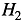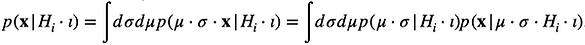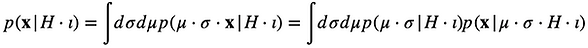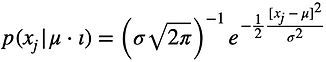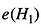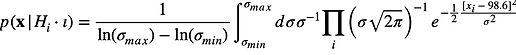th

Fig. 1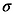Fig. 2

p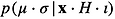bottom of page